# Beyond Mapping III

Topic 24:  Overview of Spatial Analysis and Statistics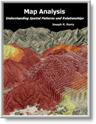Map Analysis book with

companion CD-ROM for

hands-on exercises

Moving Mapping to Analysis of Mapped Data  describes Spatial Analysis and Spatial Statistics as extensions to traditional mapping and statistics

Bending Our Understanding of Distance  uses effective distance in establishing erosion setback to demonstrate spatial analysis

discusses surface modeling to characterize the spatial distribution inherent in a data set

investigates the link between geographic space and data space for mapping data patterns

Note: The processing and figures discussed in this topic were derived using MapCalcTM software.  See www.innovativegis.com to download a free MapCalc Learner version with tutorial materials for classroom and self-learning map analysis concepts and procedures.

______________________________

(GeoWorld, December 2004, pg. 18-19)

The evolution (or is it revolution?) of GIS technology has certainly taken it well beyond traditional roles of mapping.  For over 8,000 years maps were graphic representations of physical features primarily for the purpose of navigation.  With the advent of GIS they have changed form to digital representations that are linked to databases and new processing capabilities.

Figure 1 identifies two key trends in the movement from mapping to map analysis.  Traditional GIS treats geographic space in a manner similar to our paper map legacy.  Points, lines and polygons are used to define discrete spatial objects, such as houses, streams and lakes.  In turn, these objects are linked to attributes in a database that describe their characteristics and conditions.  The result is a tremendously useful system enabling users to make complex geo-queries of the information and then map the results.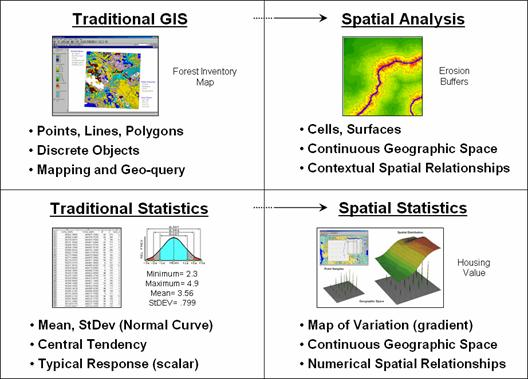Figure 1. Spatial Analysis and Spatial Statistics are extensions of traditional ways of analyzing mapped data.

Spatial Analysis extends the basic set of discrete map features of points, lines and polygons to surfaces that represent continuous geographic space as a set of contiguous grid cells.  The consistency of this grid-based structuring provides a wealth of new analytical tools for characterizing “contextual spatial relationships”, such as effective distance, optimal paths, visual connectivity and micro-terrain analysis.

In addition, it provides a mathematical/statistical framework by numerically representing geographic space.  Traditional Statistics is inherently non-spatial as it seeks to represent a data set by its typical response regardless of spatial patterns.  The mean, standard deviation and other statistics are computed to describe the central tendency of the data in abstract numerical space without regard to the relative positioning of the data in real-world geographic space.

Spatial Statistics, on the other hand, extends traditional statistics on two fronts.  First, it seeks to map the variation in a data set to show where unusual responses occur, instead of focusing on a single typical response.  Secondly, it can uncover “numerical spatial relationships” within and among mapped data layers, such as generating a prediction map identifying where likely customers are within a city based on existing sales and demographic information.

The next few columns will investigate important aspects and procedures in Spatial Analysis and Spatial Statistics through a couple of example applications in natural resources and geo-business.  Figure 2 shows the processing logic for generating a map of potential erosion for part of a large watershed.  The model assumes that erosion potential is primarily a function of terrain steepness and water flow.  Admittedly the model is simplistic but serves as a good starting point for a spatial analysis example in natural resources.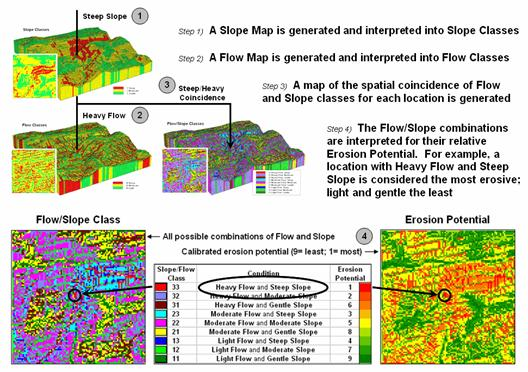Figure 2. Erosion potential is in large part dependent on the spatial combination of slope and flow.

The first step calculates a slope map that is interpreted into three classes of relative steepness—gentle (green), moderate (yellow) and steep (red) terrain.  Similarly, an accumulation flow map is generated and then interpreted into three classes of surface confluence—light (green), moderate (yellow) and heavy (red) overland flows.  The slope and flow maps are shown draped over the terrain surface to help visually verify the results.  How the slope and flow maps were derived has been discussed in previous columns focusing on procedures and algorithms involved (see Author’s Notes).  What is important for this discussion is the realization that realistic spatial considerations beyond our paper-map legacy can be derived and incorporated into map processing logic.

The third step combines the two maps into nine possible coincidence combinations and then interprets the combinations into relative erosion potential.  For example, areas with heavy flows and steep slopes have the greatest potential for erosion while areas that have light flows and gentle slopes have the least.  At this point, the calibration identifying relative erosion potential should raise some concern, but discussion of this critical step is reserved for later.  What is important at this point is a basic understanding of the spatial reasoning supporting the model’s logic.

The Erosion Potential map in figure 2 clearly shows that not all locations have the same potential to get dirt balls rolling downhill.  Given this information, a series of simple buffers based on planimetric distance would be ludicrous for protecting against sediment loading to streams.  It is common sense that a fixed distance likely would be an insufficient setback in areas of high erosion potential with heavy flows and steep intervening conditions.  Similarly the buffer would reach too far in conditions of light flow and gentle slopes.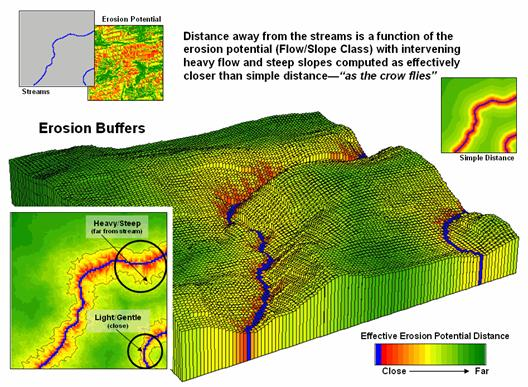Figure 3. Effective distance from streams considering erosion potential generate realistic protective buffers.

Figure 3 shows an extension of the model that reaches farther from streams under adverse erosion conditions and not as far in favorable conditions.  The result is a buffer that constricts and expands with erosion potential around the streams (see Author’s Notes).  While this “rubber-ruler” approach to establishing protective buffers isn’t part of our traditional map paradigm, it is part of real-world experience that recognizes “all buffer-feet are not the same.”

This simple example of a Spatial Analysis application illustrates how the evolution of GIS from mapping to map analysis is changing our perspective of what maps are and what we can do with them.  It also sets the stage for continued discussion of the important underlying considerations, concerns and issues involved in this new way of thinking with maps …as you will see in the next section.

___________________________

Author’s Note:  See www.innovativegis.com/basis, select the online book Map Analysis and Topic 11, Characterizing Micro-Terrain Features for discussion of slope and flow calculation; select Topic 13, Creating Variable-Width Buffers for discussion on calculating effective distance.

(GeoWorld, January 2005, pg. 18-19)

The last section introduced two dominant forces that are moving mapping toward map analysis.  Spatial Statistics is used to characterize the geographic distribution in a set of data and uncover the “numerical spatial relationships” among data layers.  Spatial Analysis extends traditional discrete map objects of point, lines and polygons to continuous surfaces that support a wealth of new analytical operations for characterizing “contextual spatial relationships.”

In describing spatial analysis, an example of generating an effective erosion buffer was used.  A comparison of simple distance (as the crow flies) and effective distance (as the crow walks) showed how a more realistic protective buffer around a stream expands and contracts depending on the erosion potential conditions surrounding the stream.  What was left unexplained was how the computer calculates the effective distance short of using a rubber ruler.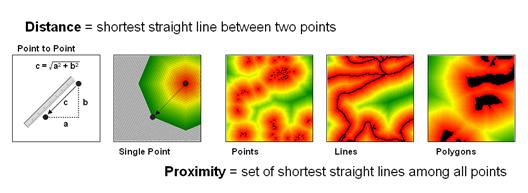Figure 1. Distance measures the space between two points while proximity identifies the spacing throughout a continuous geographic area.

The left side of figure 1 diagrams how we traditionally measure distance between two points—manually and mathematically.  We can place a ruler alongside the points and note the number of tic-marks separating them, and then multiply the map distance times the scale factor to determine the geographic distance.  Or we can calculate the X and Y differences between the points’ coordinates and use the Pythagorean Theorem to solve for the shortest straight line distance between two points (hypotenuse of a right triangle).

Spatial analysis takes the concept of distance a bit farther to that of proximity—the set of shortest straight lines among all points within a geographic area.  It is calculated as a series of distance waves that move out from one of the points.  In effect this process is analogous to nailing a ruler at the point and spinning it with the tic-marks scribing concentric circles of distance.  The distance to any point within a project area is simply its stored value indicating the number of rings away from the starting location.

If more than one feature is considered (sets of starting points, lines or polygons) the computer successively calculates proximity a starting location at a time, and keeps track of the shortest distance that is calculated for each map location.  The result is a proximity surface that identifies the distance to the closest starting point.  In figure 1 the three maps on the right identify proximity surfaces for a set of housing locations (points), the entire road network (lines) and critical habitat areas (polygons).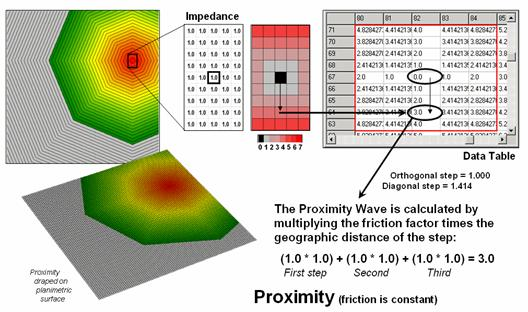Figure 2. Simple proximity considers uniform impedance as a Proximity Wave front propagates away from a starting location—“as the crow flies.”

Figure 2 illustrates the calculations for a portion of the proximity surface shown in figure 1.  The algorithm first identifies the adjoining grid cell locations that a starting location could move into (eight adjacent light grey cells).  Then it determines the geographic distance of the step as orthogonal (up/down or across = 1.000 grid space) or diagonal (slanting = 1.414) and multiples this distance times its relative impedance factor.  In the case of simple proximity the factor is constant (1.0) throughout the project area and the result is reduced to simply the geographic distance of the step.

The process is repeated for all cells within successive distance rings with the computer keeping track of the smallest distance value for each location as the waves advance (like tossing a rock into a pond).  Note that the values stored in the table increment by one in the orthogonal directions (1.0, 2.0, etc) and are adjusted for longer steps in the diagonal directions (1.414, 2.828, etc.) and off-diagonal directions (2.414, etc.) as combinations of orthogonal and diagonal steps.

Effective proximity relaxes the assumption that the relative ease of movement (impedance) is the same throughout a project area.  In the example shown in figure 3, the Proximity Wave for the first step is 1.0 (orthogonal step) times its difficulty of 8.0 (impedance factor) results in an effective distance of 8.0.  The second step has an effective distance of 3.0 (1.0 * 3.0) and combines for a total movement of 11.0 (8.0 + 3.0) from the starting location.  Note that the movement in the opposite direction is effectively a little further away ((1.0 * 7.0) + (1.0 * 5.0) = 12.0)).  In practice, half steps are used for more precise calculations (see author’s notes).

In the practical example discussed last month, a map of erosion potential was used to identify variable impedance based on slope and flow characteristics of the surrounding terrain.  Areas with high erosion potential were assigned low friction values and therefore the effective buffers reached farther than in areas of minimal erosion potential having higher friction values.  The result was a map of effective protection buffers around streams that expanded and contracted in response to localized conditions.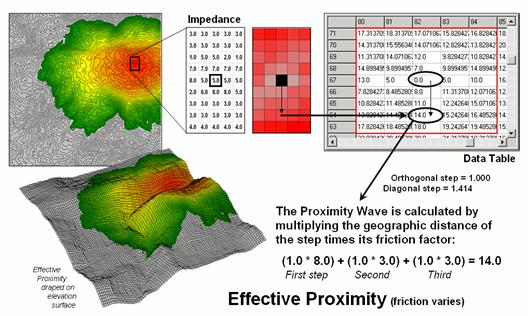Figure 3. Effective proximity considers varying impedance that changes with intervening conditions—“as the crow walks.”

Effective buffers are but one example of a multitude of new spatial analysis procedures that are altering our traditional view mapped data analysis.  Next month’s column will investigate some of the new procedures in spatial statistics that uncover numerical relationships within and among map layers.

________________________

Author’s Note:  The more exacting half-step calculation for the example is (0.5 * 5.0) + (0.5 * 8.0) + (0.5 * 8.0) + (0.5 * 3.0) + (.5 * 3.0) + (.5 * 3.0) = 15.0.  See www.innovativegis.com/basis, and select the online book Map Analysis, Topic 25, Understanding Effective Distance and Connectivity, for an in-depth discussion of how effective proximity is calculated.

Use Spatial Statistics to Map Abnormal Averages
(GeoWorld, February, 2005, pg. 18-19)

The previous couple of sections identified two dominant forces that are moving mapping toward map analysis—Spatial Analysis and Spatial Statistics.  The discussion focused on Spatial Analysis that supports a wealth of new analytical operations for characterizing “contextual spatial relationships.”   Now we can turn our attention to Spatial Statistics and how it characterizes the geographic distribution of mapped data to uncover the “numerical spatial relationships.”

Most of us are familiar with the old “bell-curve” for school grades.  You know, with lots of C’s, fewer B’s and D’s, and a truly select set of A’s and F’s.  Its shape is a perfect bell, symmetrical about the center with the tails smoothly falling off toward less frequent conditions.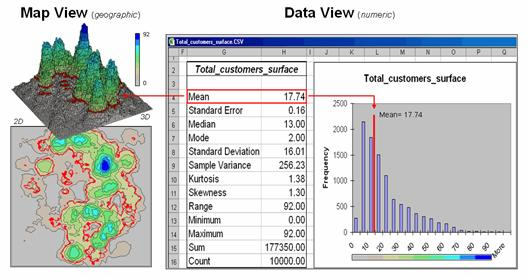Figure 1.  Mapped data are characterized by their geographic distribution (maps on the left) and their numeric distribution (descriptive statistics and histogram on the right).

However the normal distribution (bell-shaped) isn’t as normal (typical) as you might think ...in the classroom or in GIS.  The frequency distributions of mapped data tend toward the ab-normal (formally termed asymmetrical).  For example, consider the customer data shown in the figure 1.

The geographic distribution of the data is characterized in the map view by the 2D contour map and 3D surface on the left.  Note the distinct geographic pattern of the surface with bigger bumps (higher customer density) in the central portion of the project area.  As is normally the case with mapped data, the map values are neither uniformly nor randomly distributed in geographic space.  The unique pattern is the result of complex spatial processes determining where people live that are driven by a host of factors—not spurious, arbitrary, constant or even “normal” events.

Now turn your attention to the numeric distribution of the data depicted in the right side of the figure.  The data view was generated by simply transferring the grid values defining the map surface to Excel, and then applying the Histogram and Descriptive Statistics options of the Data Analysis add-in tools.  The mechanics used to plot the histogram and generate the statistics were a piece-of-cake, but the intellectual challenge is to make some sense of it all.

Note that the data aren’t distributed as a normal bell-curve, but appear shifted to the left.  The tallest spike and the intervals to its left, match the large expanse of grey values in the map view—frequently occurring values.  If the surface contained disproportionably higher value locations, there would be a spike at the high end of the histogram.  The red line in the histogram locates the mean (average) value for the numeric distribution.  The red line in the 2D and 3D maps shows the same thing, except it’s identified in the geographic distribution.

The mental exercise linking geographic space with data space is fundamental to spatial statistics and leads to important points about the nature of mapped data.  First, there isn’t a fixed relationship between the two views of the data’s distributions (geographic and data).  For example, a myriad of geographic patterns can result in the same histogram.  That’s because spatial data contains additional information—where, as well as what—and the same data summary of the “what’s” can be reflected in a multitude of spatial arrangements (“where’s).

But is the reverse true?  Can a given geographic arrangement result in different data views?  Nope, and it’s this relationship that catapults mapping and geo-query into the arena of mapped data analysis.  Traditional analysis techniques assume a functional form for the frequency distribution (histogram shape), with the standard normal (bell-shaped) being the most prevalent.

Figure 2 offers yet another perspective of the link between numeric and geographic distributions.  The upper-left inset identifies the spatial pattern formed by 16 samples of the “percent of home equity loan limit” for a small project area— ranging from 16.8 to 72.4.  The table reports the numerical pattern of the data— mean= 42.88 and standard deviation= 19.57.  The coefficient of variation is 45.6% ((19.57/42.88) * 100= 45.6%) suggesting a fairly large unexplained variation among the data values.

In a geographic context, the mean represents a horizontal plane hovering over the project area.  However, the point map suggests a geographic trend in the data from values lower than the mean in the west toward higher values in the east.  The inset in the upper-right portion of figure 2 shows a “nearest neighbor” surface generated by assigning the closest sample value to all of the other non-sampled grid locations in the project area.  While the distribution is blocky it serves as a first-order estimate of the geographic distribution you see in the point map—lower in the east and higher in the west.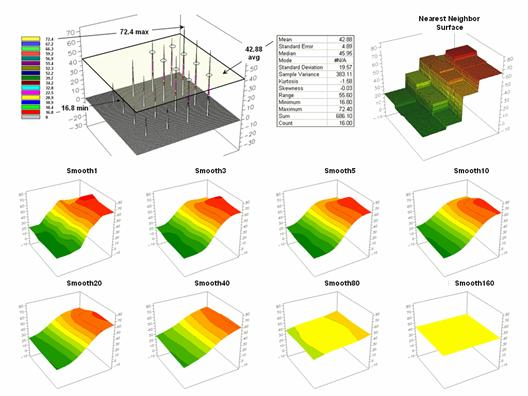Figure 2.  The spatial distribution implied by a set of discrete sample points can be estimated by iterative smoothing of the point values.

The series of plots in the lower portion of figure 2 shows the results of iteratively smoothing the blocky data.  This process repeatedly passes a “roving window” over the area that calculates the average value within quarter-mile.  The process is analogous to chipping away at the stair steps with the rubble filling in the bottom.  The first smoothing still retains most of the sharp peak and much of the angular pattern in the blocky surface.  As the smoothing progresses the surface takes on the general geographic trend of the data (Smooth10).

Eventually the surface is eroded to a flat plane— the arithmetic mean of the data.  The progressive series of plots illustrate a very important concept in surface modeling— the geographic distribution maps the variance.  Or, in other words, a map surface uses the geographic pattern in a data set to help explain the variation among the sample points.

Keep this in mind the next time you reduce a bunch of sample data to their arithmetic average and then assign that value to an entire polygon, such as a sales district, county or other administrative boundary.  In essence, this simple mapping procedure often strips out the inherent spatial information in a set of data—sort of like throwing the baby out with the bath water.

_________________________

Author’s Note:  See www.innovativegis.com/basis, and select the online book Map Analysis, Topic 2, Assessing Interpolation Results and Topic 8, Investigating Spatial Dependency.

(GeoWorld, March 2005, pg. 20-21)

The past three sections have investigated two dominant forces that are driving traditional GIS and statistics toward map analysis.  Spatial Analysis extends the representation of geographic space from discrete sets of point, line and polygonal features to map surfaces characterizing continuous space.  This grid-based structuring provides a wealth of new analytical tools that represent “contextual spatial relationships,” such as effective distance, optimal paths, visual connectivity and micro-terrain analysis.

The other force, Spatial Statistics, is used to derive “numerical spatial relationships” that map the variation in a set of data (surface modeling) and investigate the similarity among map layers to classify data patterns and develop predictive models (spatial data mining).  This final installment in the series focuses on the underlying concepts supporting data mining capabilities of analytical GIS systems.

The most fundamental notion is that geographic space and data space are interconnected.  The three maps on the left side of figure 1 depict surfaces of housing Density, Value and Age for a portion of a city.  It is important that “maps are numbers first, pictures later” and that the peaks and valleys on the surfaces are simply the graphic portrayal of actual differences in the values stored at each map location (grid cell).

The “data spear” at Point #1 identifies the housing Density as 2.1 units/ac, Value as \$407,000 and Age as 18.3 years and is analogous to your eye noting a color pattern of green, red, and green.  The other speared location locates a very dissimilar data pattern with Density=  4.8 units/ac, Value= \$190,000 and Age= 51.2 years—or as your eye sees it, a color pattern of red, green and red.

The right side of figure 1 depicts how the computer “sees” the data patterns for the two locations in three-dimensional data space.  The three axes defining the extent of the box correspond to housing Density (D), Value (V) and Age (A).  The floating balls represent data patterns of the grid cells defining the geographic space—one “floating ball” (data point) for each grid cell.  The data values locating the balls extend from the data axes—2.41, 407.0 and 18.3 for Point #1.  The other point has considerably higher values in D and A with a much lower V values (4.83, 51.2 and 190.0 respectively) so it plots at a very different location in data space.

Figure 1.  Conceptually linking geographic space and data space.

The fact that similar data patterns plot close to one another in data space with increasing distance indicating less similarity provides a foothold for mapping numerical relationships.  For example, a cluster map divides the data into groups of similar data patterns as schematically depicted in figure 2.

As noted before the floating balls identify the data patterns for each map location (geographic space) plotted against the P, K and N axes (data space).  For example, the tiny green ball in the upper-left corner depicts a map location in the fairly wealthy part of town (low D, high V and low A).  The large red ball appearing closest to you depicts a location in the less affluent part (high D, low V and high A).

It seems sensible that these two extreme responses would belong to different data groupings (clusters 1 and 2) with the red area locating less wealthy locations while the green area identifies generally wealthier sections.  In a similar fashion, the project area can be sub-divided into three and four clusters identifying more detailed data pattern groupings.

Figure 2.  Data patterns for map locations are depicted as floating balls in data space that can be grouped into clusters of similar patterns based on their data distances.

While the specific algorithm used in clustering is beyond the scope of this discussion, it suffices to note that data distances between the floating balls are used to identify cluster membership—groups of balls that are relatively far from other groups and relatively close to each other form separate data clusters.  Other techniques, such as map similarity and regression, use the link between geographic and data space to characterize spatial relationships and develop prediction maps.

The realization that mapped data can be expressed in both geographic space and data space is paramount to a basic understanding of how a computer analyses numerical interrelationships among mapped data.  Geographic space uses coordinates, such as latitude and longitude, to locate things in the real world.  The geographic expression of the complete set of measurements depicts their spatial distribution in familiar map form.

Data space, on the other hand, is a bit less familiar.  While you can’t stroll through data space you can conceptualize it and write algorithms that analyze it … as well as imagine plenty of potential of applications from geo-business to natural resources.  Coupling the power of Spatial Statistics with that of Spatial Analysis takes traditional statistics and GIS to a whole new plane.

____________________________

Author’s Note:  See www.innovativegis.com/basis, and select the online book Map Analysis, Topic 10, Analyzing Map Similarity and Zoning and Topic 16, Characterizing Patterns and Relationships.